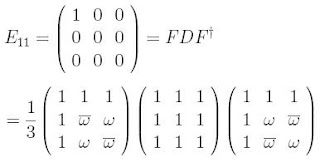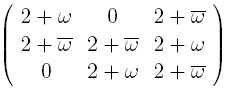occasional meanderings in physics' brave new world

Saturday, March 27, 2010

M Theory Lesson 308

Any elementary matrix, denoted $E_{ij}$, can be expressed as a Fourier transform of the democratic matrix.In the $3 \times 3$ case, the nine choices for $F$ give the $9$ elementary matrices. Thus any matrix at all may be expressed as a combination of such Fourier transforms. For example, the circulant permutation $(231)$ uses the three Fourier matrices that sum toA general $1$-circulant is therefore a combination of three transforms, each of this form. An alternative choice of phases for $(231)$ would have given uswhich is a bit more reminiscent of CKM symmetries. Since the tribimaximal mixing matrix may be expressed as a product $F_3 F_2$, one hopes that the CKM matrix is also easily written in terms of a natural transform.L. Riofrio said...